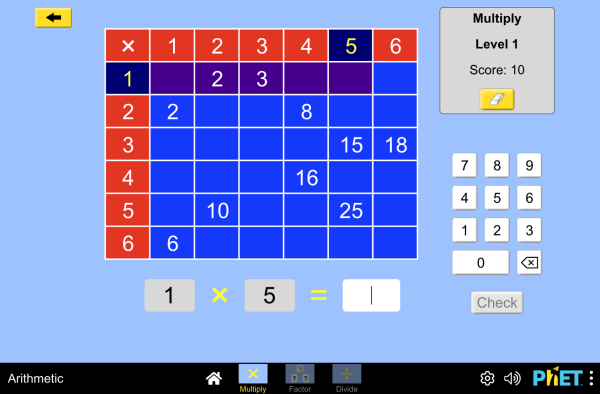# 四则运算下载嵌入代码 关闭 嵌入模拟程序控制 你可以通过改变嵌入的代码改变程序的宽度和高度 插入一张图片，当点击这个图片时启动仿真程序。初始仿真程序 以及翻译

• 增殖
• 除法
• 因式分解

### 示范 学习目标

• 解释乘法表是如何促进乘法、因子以及分解的理解。提高乘法、因子分解、除法的精确度。

### 标准对齐

#### Common Core - Math

3.OA.A.1
Interpret products of whole numbers, e.g., interpret 5 × 7 as the total number of objects in 5 groups of 7 objects each. For example, describe a context in which a total number of objects can be expressed as 5 × 7.
3.OA.A.2
Interpret whole-number quotients of whole numbers, e.g., interpret 56 ÷ 8 as the number of objects in each share when 56 objects are partitioned equally into 8 shares, or as a number of shares when 56 objects are partitioned into equal shares of 8 objects each. For example, describe a context in which a number of shares or a number of groups can be expressed as 56 ÷ 8.
3.OA.A.4
Determine the unknown whole number in a multiplication or division equation relating three whole numbers. For example, determine the unknown number that makes the equation true in each of the equations 8 × ? = 48, 5 = _ ÷ 3, 6 × 6 = ?
3.OA.B.5
Apply properties of operations as strategies to multiply and divide.2 Examples: If 6 × 4 = 24 is known, then 4 × 6 = 24 is also known. (Commutative property of multiplication.) 3 × 5 × 2 can be found by 3 × 5 = 15, then 15 × 2 = 30, or by 5 × 2 = 10, then 3 × 10 = 30. (Associative property of multiplication.) Knowing that 8 × 5 = 40 and 8 × 2 = 16, one can find 8 × 7 as 8 × (5 + 2) = (8 × 5) + (8 × 2) = 40 + 16 = 56. (Distributive property.)
3.OA.B.6
Understand division as an unknown-factor problem. For example, find 32 ÷ 8 by finding the number that makes 32 when multiplied by 8.
3.OA.C.7
Fluently multiply and divide within 100, using strategies such as the relationship between multiplication and division (e.g., knowing that 8 × 5 = 40, one knows 40 ÷ 5 = 8) or properties of operations. By the end of Grade 3, know from memory all products of two one-digit numbers.
3.OA.D.9
Identify arithmetic patterns (including patterns in the addition table or multiplication table), and explain them using properties of operations. For example, observe that 4 times a number is always even, and explain why 4 times a number can be decomposed into two equal addends.

### 教师指南仿真程序控制概述,简化模型,学生视角的思考 ( PDF ).

### 教师提交的活动

Juego de Aritmética (Basado en Indagación)Trish Loeblein, traducido por Diana López 高中

Games Remote Lesson ideasTrish Loeblein 初中

K-5

Remote

Arithmetic Games (Inquiry Based)Trish Loeblein K-5

Remote

Arithmetic (Multiply)Philippe Guegan 初中 指导 数学
SECUNDARIA: Alineación PhET con programas de la SEP México (2011 y 2017)Diana López 高中

PREPARATORIA: Alineación de PhET con programas de la DGB México (2017)Diana López 高中

PRIMARIA: Alineación con programas de la SEP México (2011 y 2017)Diana López 初中
K-5

Preguntas de razonamiento para todas las simulaciones HTML5Diana López 高中

K-5

How do PhET simulations fit in my middle school program?Sarah Borenstein 初中 其它 化学

Fixação da técnica de assertividade Francisco Aislan da Silva Freitas, Gilvandenys Leite Sales K-5 实验室 数学
Atividade de Aritmética Ramon Albuquerque 初中

MS and HS TEK to Sim Alignment Elyse Zimmer 高中

How are products and factors related? Seda Serpin K-5 实验室

Math facts - Using the Multiplication Chart Tapan Sarkar 初中 实验室 数学
Discovering divisibility rules Stacy Larson 高中

### HTML5 程序：

iOS 8+ Safari

Android:

Chromebook:

Windows 系统:

Macintosh 系统:
OS 10.9.5 或之后的系统版本，Safari 9+版本，或者火狐浏览器，谷歌浏览器的最新版本均支持我们的程序。

Linux 系统:

### Java and Flash 程序：

Java 与 Flash程序可以在PC端，mac端，及linux系统上运行。具体的系统配置要求如下：

Windows 系统:
Windows 10, 8.1, 7

Macintosh 系统:
Mac OS X 10.9.5+

64位浏览器（例如Safari，火狐，谷歌等）

Linux 系统:
Oracle Linux 5.5+1
Oracle Linux 6.x (32位), 6.x (64位)2
Oracle Linux 7.x (64位)2
Red Hat Enterprise Linux 5.5+1, 6.x (32位), 6.x (64位)2
Ubuntu Linux 12.04 LTS, 13.x
Suse Linux Enterprise Server 10 SP2+, 11.x

• John Blanco (developer)
• Bryce Gruneich
• Karina K.R. Hensberry
• Trish Loeblein
• Ariel Paul
• Kathy Perkins